# matplotlib-plt.plot用法1147-柳同学

## 热门标签

,

matplotlib.pyplot.plot(*args, scalex=True, scaley=True, data=None, **kwargs)
将x,y绘制为线条或标记
参数：
x, y：数据点的水平/垂直坐标。x值是可选的，默认为range（len（y））。通常，这些参数是
一维数组。它们也可以是标量，也可以是二维的（在这种情况下，列代表单独的数据集）。
这些参数不能作为关键字传递。
fmt：格式字符串，格式字符串只是用于快速设置基本行属性的缩写。所有这些以及更多这些都可以
通过关键字参数来控制。此参数不能作为关键字传递。
data:具有标签数据的对象。如果提供，请提供标签名称以在x和y中绘制

其他参数：
scalex, scaley：这些参数确定视图限制是否适合数据限制。这些值将传递到autoscale_view
**kwargs：kwarg用于指定属性，例如线标签（用于自动图例），线宽，抗锯齿，标记面颜色等
如果使用一个plot调用生成多条线，则kwarg应用于所有这些线。

>>> plot(x, y)        # plot x and y using default line style and color
>>> plot(x, y, 'bo')  # plot x and y using blue circle markers
>>> plot(y)           # plot y using x as index array 0..N-1
>>> plot(y, 'r+')     # ditto, but with red plusses

>>> plot(x, y, 'go--', linewidth=2, markersize=12)
>>> plot(x, y, color='green', marker='o', linestyle='dashed',
...      linewidth=2, markersize=12)

>>> plot('xlabel', 'ylabel', data=obj)

>>> plot(x1, y1, 'bo')
>>> plot(x2, y2, 'go')

>>> plot(a, a[1:])

>>> plot(x1, y1, 'g^', x2, y2, 'g-')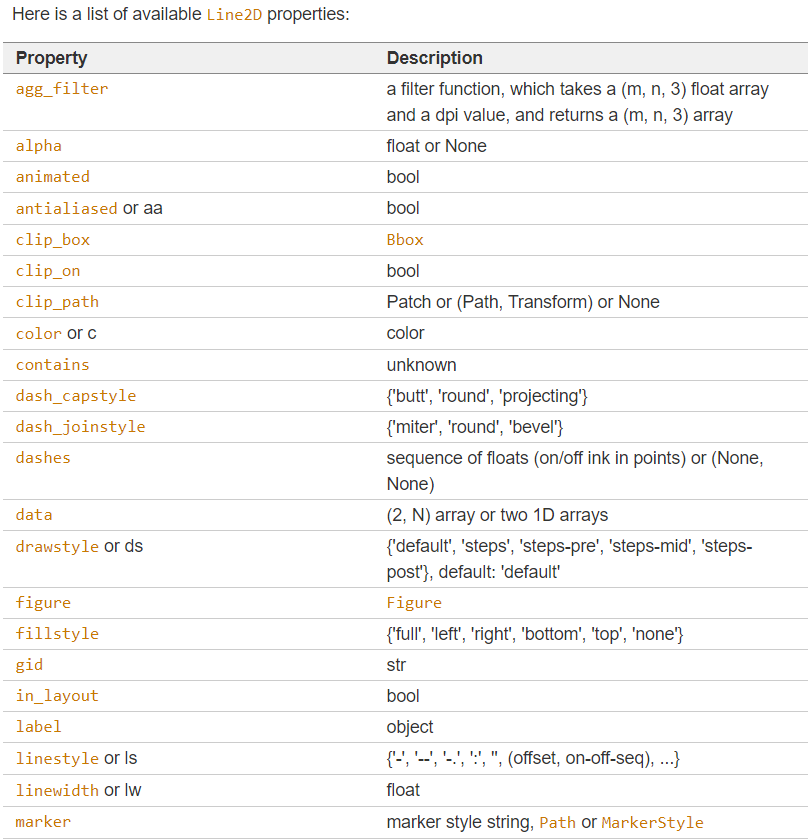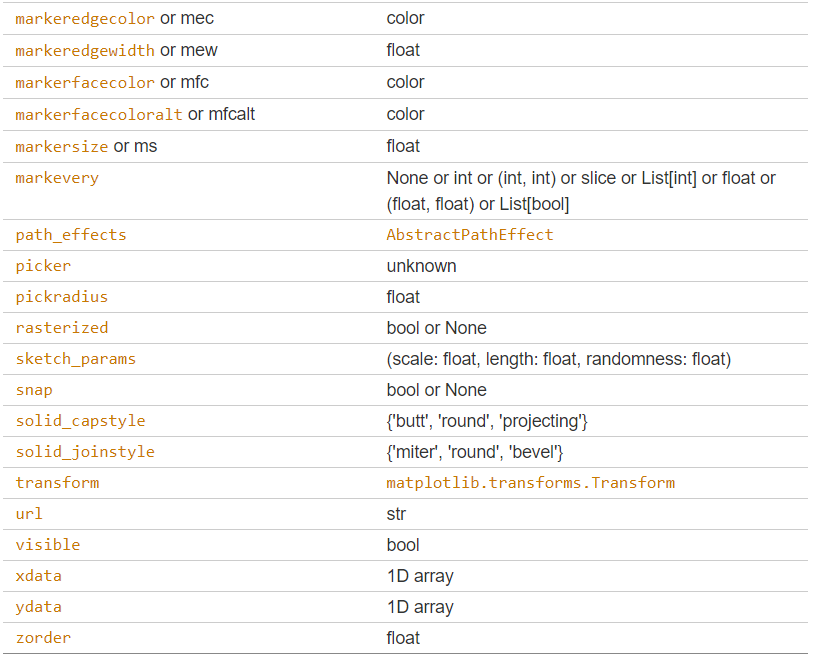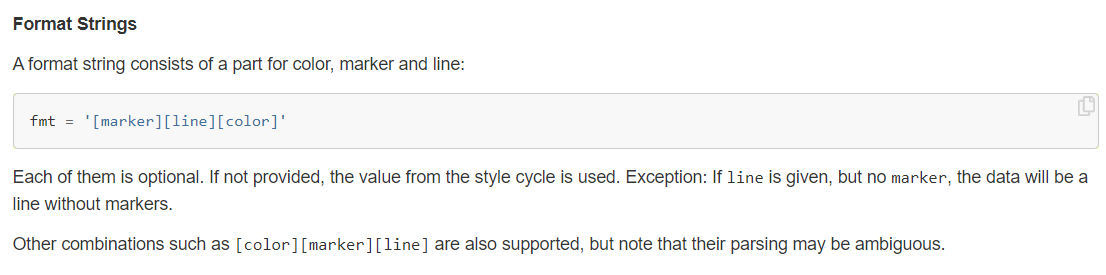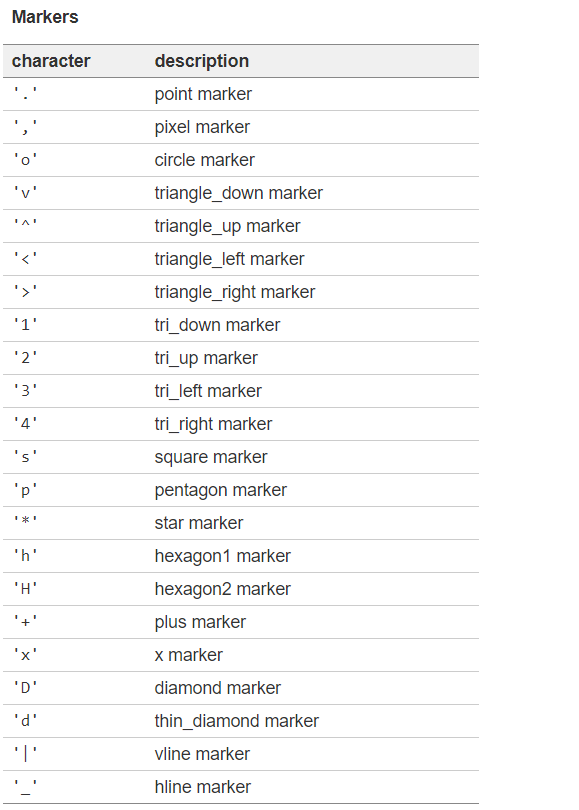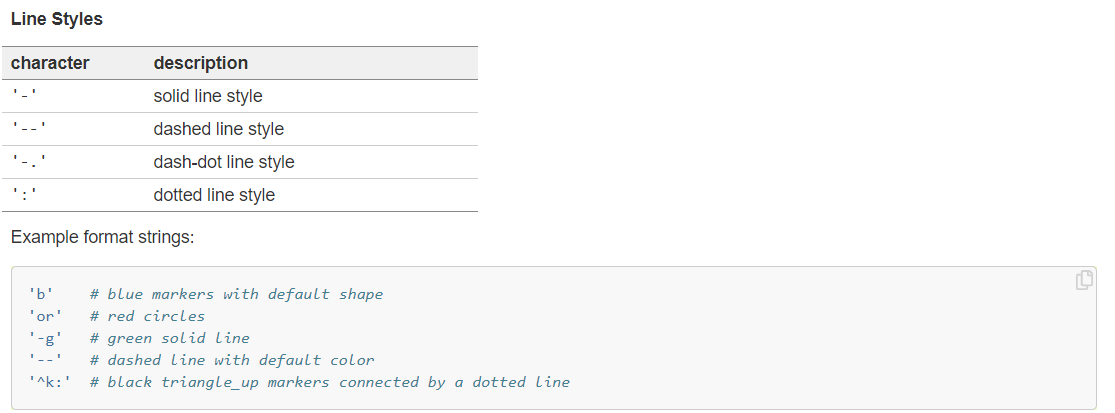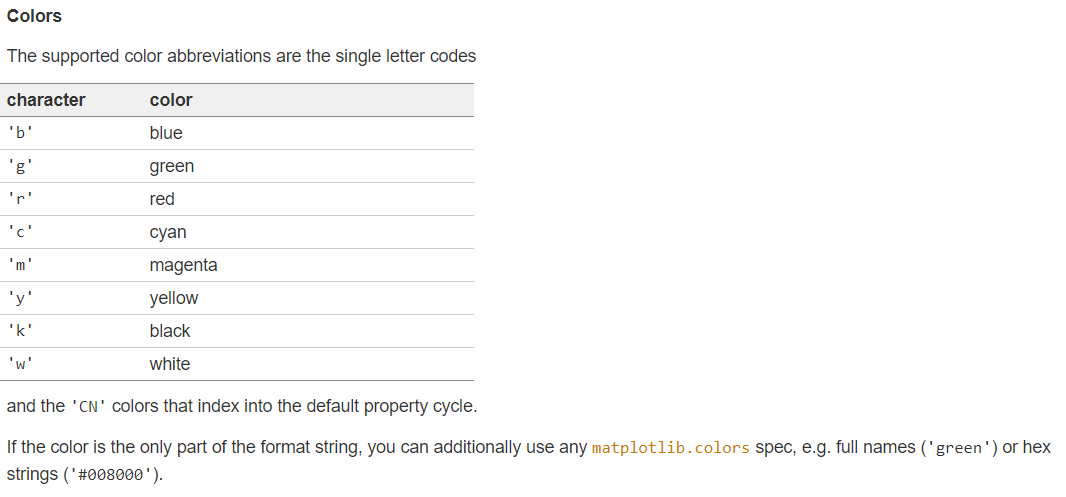### 评论 抢沙发### 觉得文章有用就打赏一下文章作者

#### 支付宝扫一扫打赏#### 微信扫一扫打赏Vieu3.3主题Q Q 登 录